# Trigonometry

GCSELevel 4-5Level 6-7Cambridge iGCSEEdexcel iGCSE

## Trigonometry

Trigonometry is the study of the relationship between the angles of a triangle and its lengths. We have functions that can tell us these relationships, the primary ones we will be looking at are the sine function, the cosine function, and the tangent function. We abbreviate these to sin, cos and tan

The trigonometric functions are functions of angles.

## SOHCAHTOA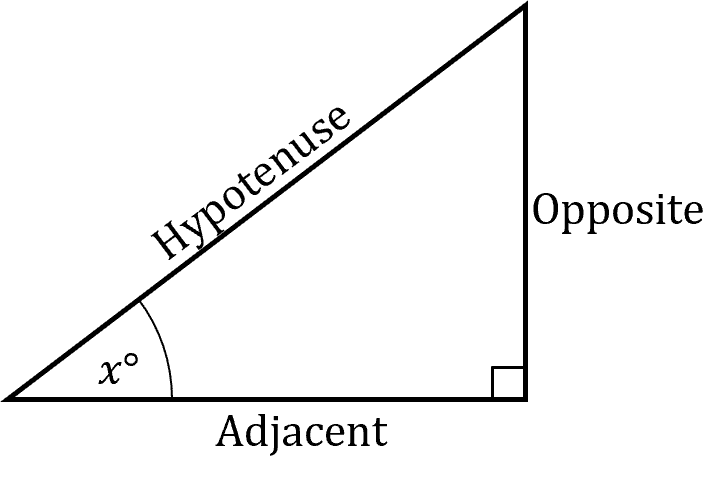SOHCAHTOA is a memory device for recalling how the trigonometric functions relate to the lengths of a right angle triangle.

From the angle $x$, the triangle has three relative sides.

Hypotenuse – The side opposite the right angle.

Opposite – The side opposite the $x$ angle.

Adjacent – The side next to the $x$ angle.

This is for when $x$ is not the $90\degree$ angle.

Then for $x$

$\textcolor{#f43364}{\text{sin}}(x) = \dfrac{\textcolor{#7cb447}{\textbf{Opposite}}}{\textcolor{#2730e9}{\textbf{Hypotenuse}}}$

$\textcolor{#00d865}{\text{cos}}(x) = \dfrac{\textcolor{#bd0000}{\textbf{Adjacent}}}{\textcolor{#2730e9}{\textbf{Hypotenuse}}}$

$\textcolor{#10a6f3}{\text{tan}}(x) = \dfrac{\textcolor{#7cb447}{\textbf{Opposite}}}{\textcolor{#bd0000}{\textbf{Adjacent}}}$

Abbreviating $\text{sin}(x)$ to $\text{S}$, $\text{cos}(x)$ to $\text{C}$, $\text{tan}(x)$ to $\text{T}$, Opposite to $\text{O}$Adjacent to $\text{A}$ and Hypotenuse to $\text{H}$, we get

 $\text{S} = \dfrac{O}{H}$ $\text{C} = \dfrac{A}{H}$ $\text{T} = \dfrac{O}{A}$

Which is where SOHCAHTOA comes from.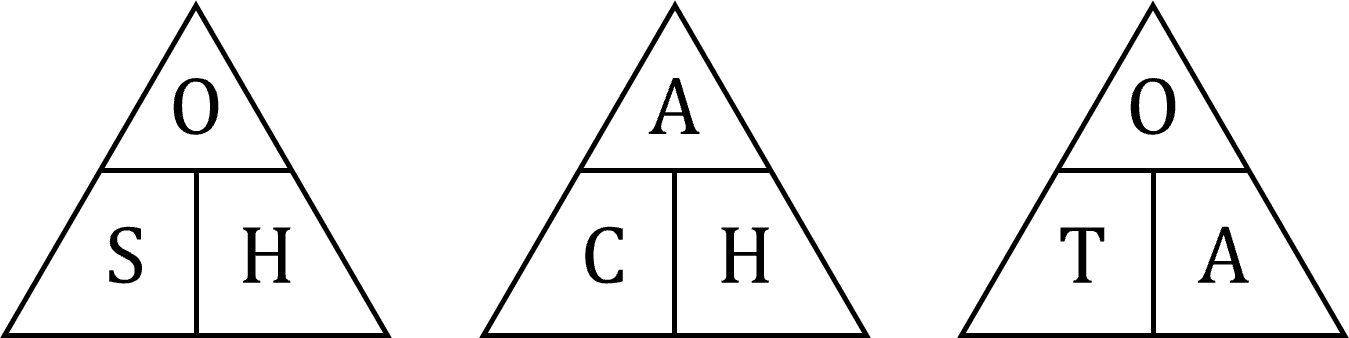Level 4-5GCSECambridge iGCSEEdexcel iGCSE

## Angles of Elevation and Depression

When looking straight forward, your eyeline is parallel to the horizon. When you want to look at something above your eyeline, you look up. The angle at which you look up is called the angle of elevation.

Similarly, if you want to view something below your eyeline, you look down. The angle to which you look down is called the angle of depression.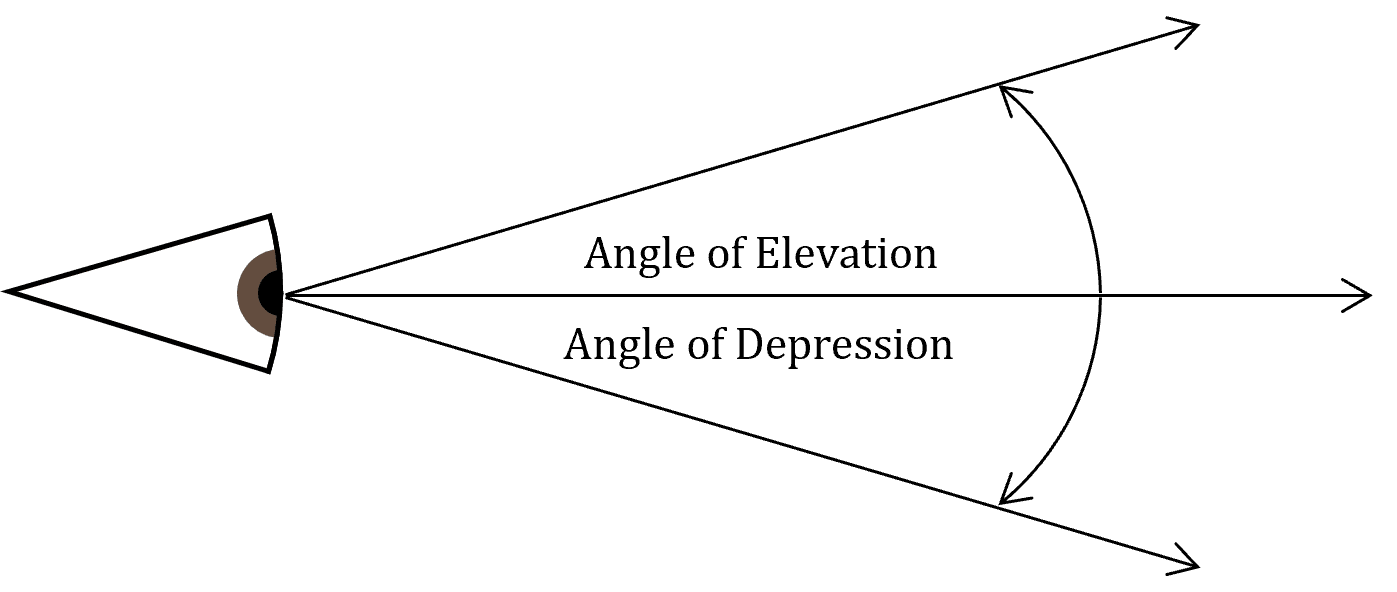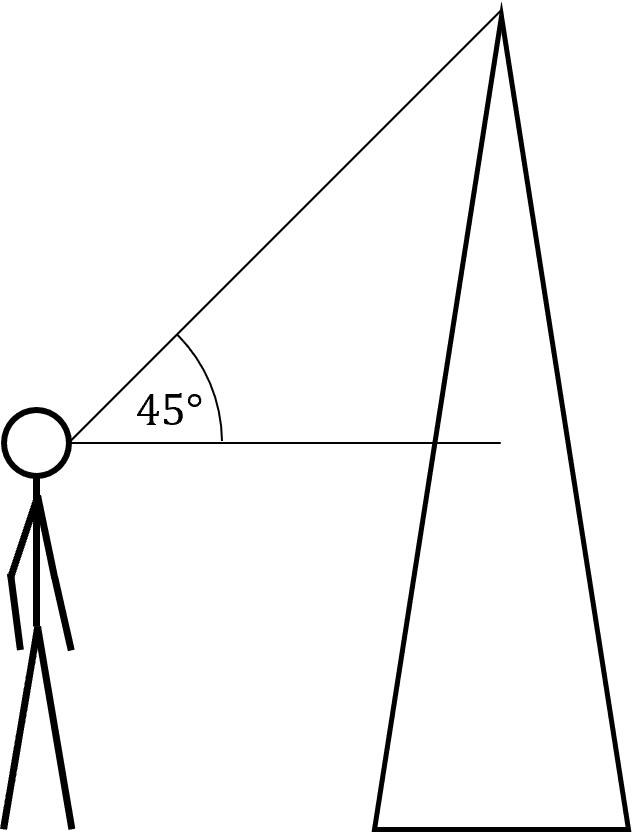We can use these angles to construct right angled triangles, on which we can do trigonometry. For example, if you were standing $308$ meters from the base of the Shard, and you knew that your eye level was $1.6$ meters from the ground, if your angle of elevation is $45\degree$ then you can use this to calculate the height of the Shard.

You have the angle $45\degree$, and the adjacent length of $308 \text{ m}$. So recalling SOHCAHTOA

$\text{tan}(x) = \dfrac{\text{Opposite}}{\text{Adjacent}}$

So, using our calculator for $\text{tan}(45) = 1$ we have

$1 = \dfrac{\text{Opposite}}{308}$

$\text{Opposite} = 308 \text{ m}$

We must remember that it is $308$ plus our eye level, giving us a height for the shard of $309.6 \text{ m}$.

The same process can be used with an angle of depression to find the depth of things below our eyeline.

Level 6-7GCSECambridge iGCSEEdexcel iGCSE

## Shortest Distance

For a point $P$ and a line $L$, the shortest line from $P$ to $L$ is perpendicular to $L$.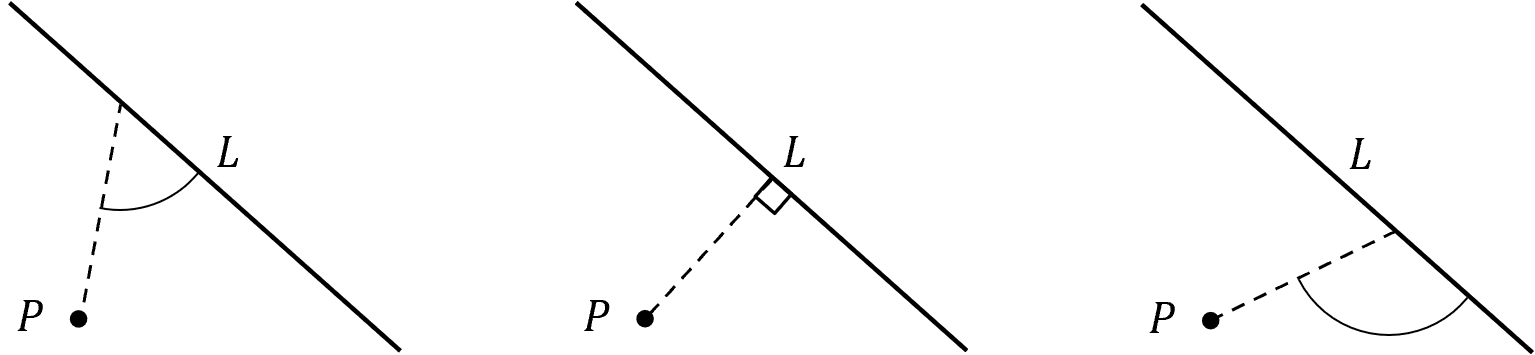This must be the shortest length between $P$ and $L$ as any line connecting $P$ and $L$ at any other angle is the hypotenuse to a triangle with the perpendicular line. As the hypotenuse is always the largest side of a right angled triangle, the perpendicular line must be the shortest possible distance from $P$ to $L$.

We may use this fact to calculate, using SOHCAHTOA, the distances between points and lines.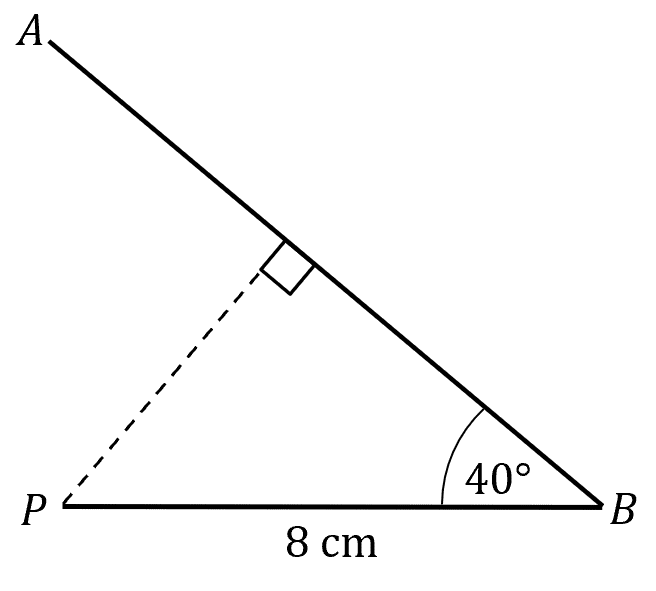Example: If the line $AB$ passes though points $A$ and $B$ whilst line $PB = 8 \text{ cm}$ and $\angle PBA = 40 \degree$ then we can work out the shortest distance from $P$ to line $AB$.

As the shortest distance is the line perpendicular to $AB$ from $P$ to the line $AB$, we can construct a right angled triangle, with the angle $40 \degree$ and the hypotenuse of $PB = 8 \text{ cm}$. Which would leave us looking for the opposite side.

By SOHCAHTOA

$\text{Opposite} = \text{sin}(x) \times \text{Hypotenuse}$

with our values

$\text{Shortest distance}=\text{sin}(40) \times 8$

$\text{Shortest distance}=5.1\text{ cm}$

to $1$ decimal place.

Level 6-7GCSECambridge iGCSE

## Example 1: Finding a Side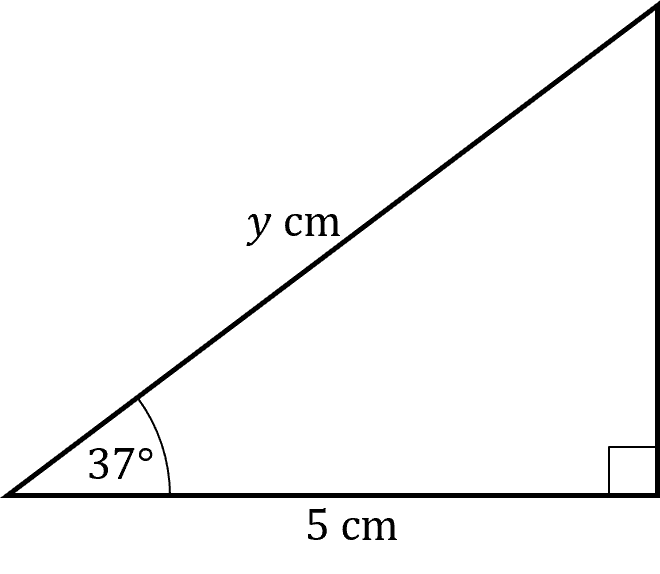Find the missing length $y$ to $1$ decimal place.

[2 marks]

First, we can see that we have the angle $37 \degree$ and it’s adjacent length $5 \text{ cm}$. We are looking for the length of the hypotenuse, so with SOHCAHTOA, we know

$\text{cos}(x) = \dfrac{\text{adjacent}}{\text{hypotenuse}}$

substituting in our values

$\text{cos}(37) = \dfrac{5}{y}$

$y = \dfrac{5}{\text{cos}(37)}$

Using our calculators we get

$y = 6.260 \dots$

So the length of the hypotenuse is $6.3 \text{ cm}$ to $1$ decimal place.

Level 4-5GCSECambridge iGCSEEdexcel iGCSE

## Example 2: Finding an Angle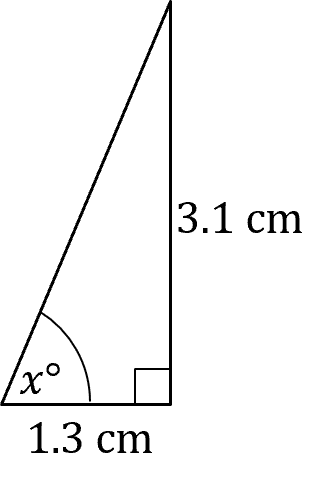Find the missing angle $x$ to the nearest degree.

[2 marks]

We are looking for an angle $x$ and we have it’s adjacent length $1.3 \text{ cm}$ and it’s opposite length $3.1 \text{ cm}$. Using SOHCAHTOA we have

$\text{tan}(x) = \dfrac{\text{opposite}}{\text{adjacent}}$

Substituting our values in

$\text{tan}(x) = \dfrac{3.1}{1.3} = \dfrac{31}{13}$

We must use the inverse function of $\text{tan}$, this is the $\text{tan}^{-1}$ function. You should be able to find this on a calculator. The inverse function is defined such that:

$\text{tan}^{-1}(\text{tan}(x)) = x$

So substituting in $\text{tan}(x) = \dfrac{31}{13}$

$x =\text{tan}^{-1} \left( \dfrac{31}{13} \right) = 67.249 \dots$

So $x$ to the nearest degree is $67\degree$.

Level 4-5GCSECambridge iGCSEEdexcel iGCSE

## Example 3: Angle of Depression

Tower $A$ is $200 \text{ m}$ away from tower $B$. From the peak of tower $A$, the angle of depression when looking down at the peak of tower $B$ is $24 \degree$, how much shorter is tower $B$ than tower $A$, to the nearest metre?

[4 marks]

We can construct the right angled triangle to find the height difference.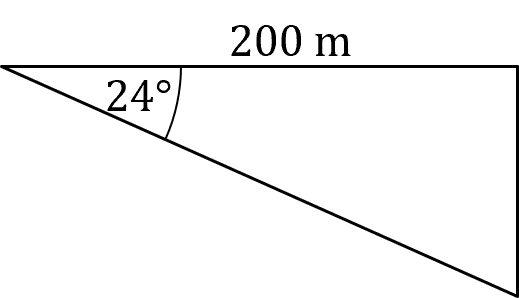Now we have a right angled triangle with an angle of $24 \degree$, the adjacent length is $200 \text{ m}$ and the opposite length is what we are looking for. From SOHCAHTOA we have

$\text{tan}(x) = \dfrac{\text{opposite}}{\text{adjacent}}$

Substituting in the values we have gives us

$\text{tan}(24) = \dfrac{\text{opposite}}{200}$

$\text{opposite} = 200 \times\text{tan}(24)$

$\text{opposite} = 89.045 \dots \text{ m}$

So tower $A$ is $89 \text{ m}$ taller than tower $B$.

Level 6-7GCSECambridge iGCSEEdexcel iGCSE

## Trigonometry Example Questions

We have a known angle and the hypotenuse. We are looking for the adjacent side. So using SOHCAHTOA we know

$\text{cos}(x) = \dfrac{\text{adjacent}}{\text{hypotenuse}}$

So putting in our values

$\text{cos}(64) = \dfrac{y}{5.2}$

$y = \text{cos}(64) \times 5.2 = 2.279 \dots$

So the missing length is $2.3 \text{ mm}$ to $1$ decimal place.

From the angle we are looking for we have its adjacent side and its opposite side. From SOHCAHTOA we have

$\text{tan}(x) = \dfrac{\text{opposite}}{\text{adjacent}}$

Substituting in our values

$\text{tan}(x) = \dfrac{3}{3.3}$

So using $\text{tan}^{-1}$ to find $x$

$x = \text{tan}^{-1} \left( \dfrac{3}{3.3} \right)$

$x = 42.27 \dots$

So the missing angle is $42 \degree$ to the nearest degree.

If Nina’s house is  $6.5 \text{ m}$ and her eyeline is $1.5 \text{ m}$ off the ground, then when she looks up at her house, she is only looking $5 \text{ m}$ up. Given this and the angle of elevation of $25 \degree$, we can construct a right angled triangle.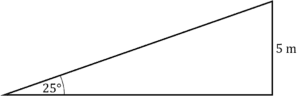Now to find out how far away from her house she is, we can use SOHCAHTOA. We have an angle and it’s opposite side, while we are looking for the adjacent side.

$\text{tan}(x) = \dfrac{\text{opposite}}{\text{adjacent}}$

So using our values

$\text{tan}(25) = \dfrac{5}{\text{adjacent}}$

$\text{adjacent} = \dfrac{5}{\text{tan}(25)} = 10.722 \dots$

So Nina is standing $10.7 \text{ m}$ away from her house.

If the distance from jack to the beach is $25 \text{ m}$ and it has an angle of depression of $32 \degree$ then we can construct a right angled triangle.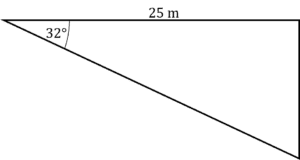Now we want to find the opposite side to the angle and we have the adjacent angle. Using SOHCAHTOA we know

$\text{tan}(x) = \dfrac{\text{opposite}}{\text{adjacent}}$

Substituting our values in

$\text{tan}(32) = \dfrac{\text{opposite}}{25}$

$\text{opposite} = 25 \times \text{tan}(32) = 15.621 \dots$

Jack is $15.6 \text{ m}$ high.

The shortest distance from $C$ to $AB$ is the length of the line from $C$ to $AB$ that is perpendicular to $AB$

So we construct a right angled triangle where $BC$ is the hypotenuse and the perpendicular line from $C$ to $AB$ is the opposite side to the angle $\angle ABC$.

Using SOHCAHTOA

$\text{Opposite} = \text{sin}(x) \times \text{Hypotenuse}$

With our values

$\text{Shortest Distance}= \text{sin}(28) \times 3.5$

$\text{Shortest Distance}= 1.64 \text{ cm}$

To $2$ decimal place.

## You May Also Like...### MME Learning Portal

Online exams, practice questions and revision videos for every GCSE level 9-1 topic! No fees, no trial period, just totally free access to the UK’s best GCSE maths revision platform.

£0.00